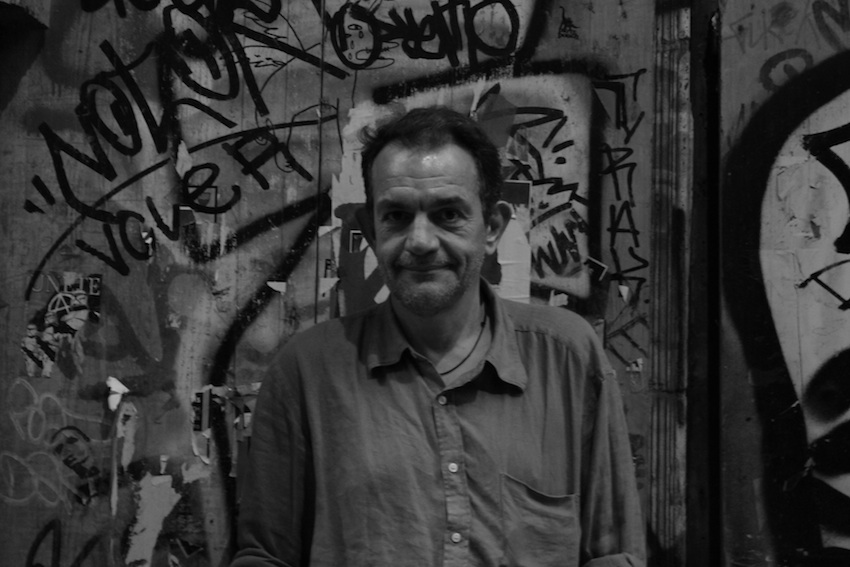Vangelis Th. PaschosProfessor of Computer Science at the University Paris-Dauphine

Member of the research group: "Combinatorial Optimization, Algorithms" of the LAMSADE

Research interests:

Complexity theory

Worst-case complexity of exact algorithms for NP-hard problems

Approximation of NP-hard optimization problems

Polynomial approximability

Moderately exponential, subexponential and parameterized approximation

Algorithmics in dynamic environments (Probabilistic combinatorial optimization, On-line algorithms, Reoptimization)